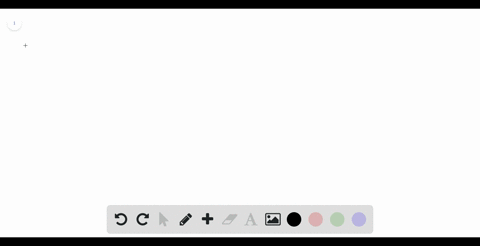### Determine a region whose area is equal to the giv…

00:52University of California, Berkeley
Problem 24

Determine a region whose area is equal to the given limit. Do not evaluate the limit.

$\displaystyle \lim_{n \to \infty} \sum_{i = 1}^{n} \frac{3}{n} \sqrt{1 +\frac{3i}{n}}$

This sum represents the area under the curve $y=\sqrt{x}$ and above $x$ -axis in
the interval $x \in[1,4]$

## Discussion

You must be signed in to discuss.

## Video Transcript

The first thing we know is that Delta Axe is B minus over on which we know that when we compare, we can see that Delta acts this three over on is one still toe axe is B minus over on and we know that it's b minus one over at is equivalent to three over and then we can get the value to be is be his force A is one b is for we know f of X escort of ex, obviously from 1 to 4 squirt of axe DX. So what this means is this Some represents the area under the curve y equals quarterbacks and above the X axis in the interval from 1 to 4 is given from our A and B, which we calculated.